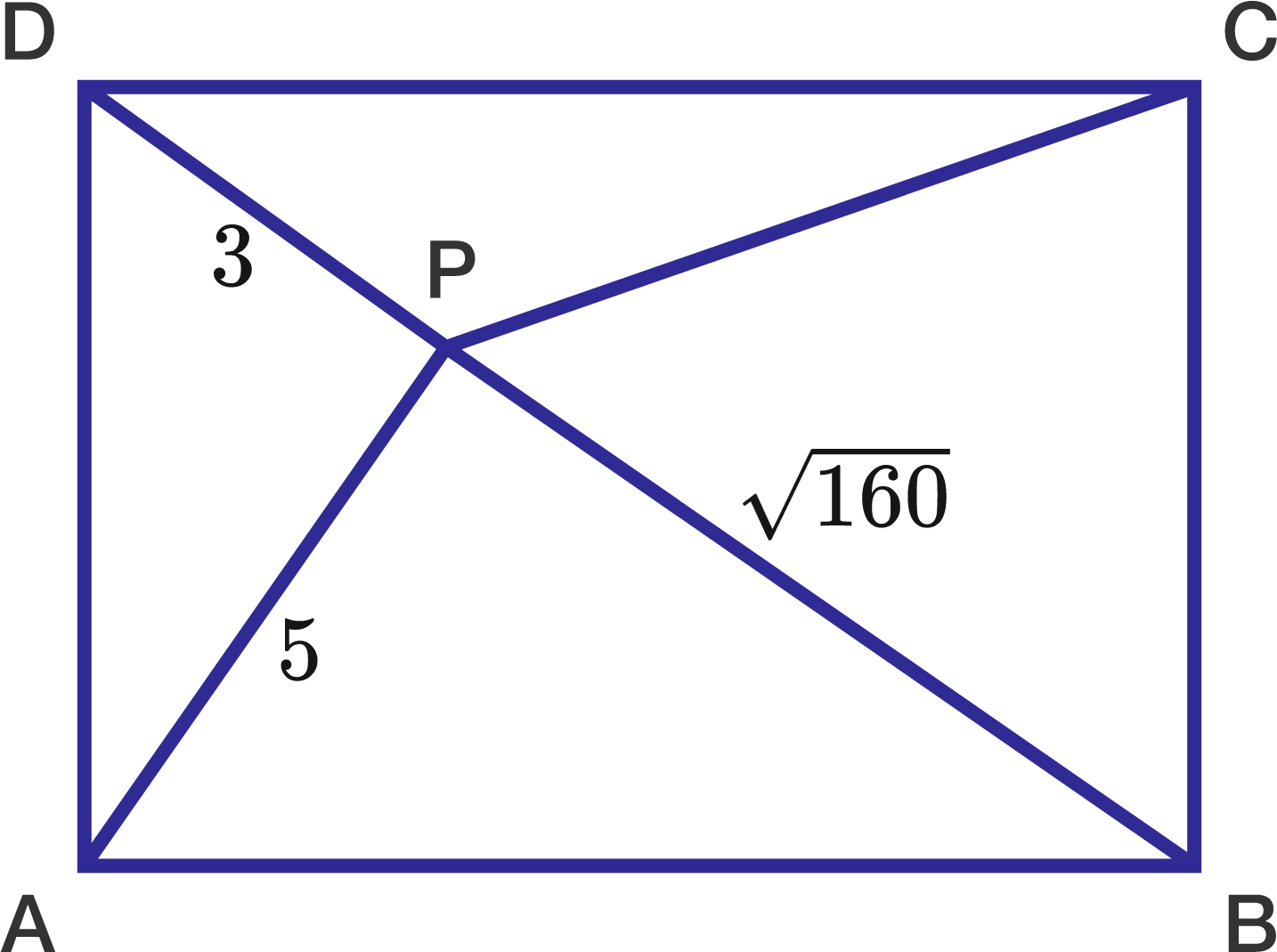# Rectangle and a random point

Geometry Level 3The above shows a rectangle $ABCD$ with $P$ as a point in the interior of this rectangle.

We are given the distances $DP = 3, AP = 5, BP = \sqrt{160}$.

Find the length of $CP$.

###### Try another problem on my set, Let's Practice
×

Problem Loading...

Note Loading...

Set Loading...